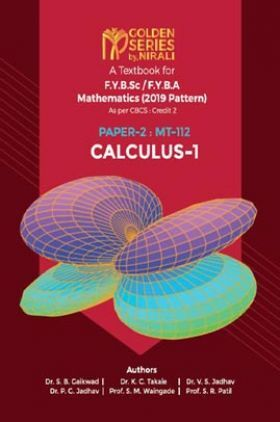# Calculus - I

( 53 customers rating )
940 Views
MRP : ₹125.00
Price : ₹83.60
You will save : ₹41.40 after 33% Discount
Inclusive of all taxes

Save extra with 2 Offers

Get ₹ 50

Instant Cashback on the purchase of ₹ 400 or above

Product Specifications

 Publisher Nirali Prakashan All B.A - Bachelor of Arts books by Nirali Prakashan ISBN 9789389108637 Author: Dr. S. B. Gaikwad, Dr. K. C. Takale, Dr. V. S. Jadhav, Dr. P. G. Jadhav, Prof. S. M. Waingade, Prof. S. R. Patil Number of Pages 133 Edition First Edition Available Available in all digital devicesCalculus - I by Dr. S. B. Gaikwad, Dr. K. C. Takale, Dr. V. S. Jadhav, Dr. P. G. Jadhav, Prof. S. M. Waingade, Prof. S. R. Patil
Book Summary:

This book is based on a course Calculus-I. We write this book as per the revised syllabus of F.Y. B.Sc. Mathematics, revised by Savitribai Phule Pune University, Pune, implemented from June 2019. Calculus is the most useful subject in all of mathematics and it is used extensively in applied mathematics and engineering.

In Chapter 1, we present the real number system is de ned as a nonempty set R together with two algebraic operations, addition and multiplication, and an ordering less than that collectively satisfy three sets of axioms: the eld axioms, the order axioms and the completeness axiom. Furthermore, we prove properties of real numbers and solve some examples.

In the second Chapter, we study the sequence of real numbers, limit of a sequence and their con- vergence. Furthermore, we study bounded sequence, monotone sequence, also, we prove theorems to related topics and solve some examples.

The third Chapter deals with the concept of to plot the graph of functions, nd its natural domain and range. Furthermore, we de ne limit of a function and prove some theorems on limits. Also, we de ne sequential criterion of limit, limit at in nity and solve some examples.

In fourth Chapter, we study the continuity of a function at a point and in an interval. Also, we discuss the sequential criterion of continuity of the function. We prove the theorems to related topics and solve some examples.

Audience of the Book :
This book Useful for B.Sc & BA Students.
Table of Content:

1. Real Numbers

2. Sequences

3. Limits

4. Continuity